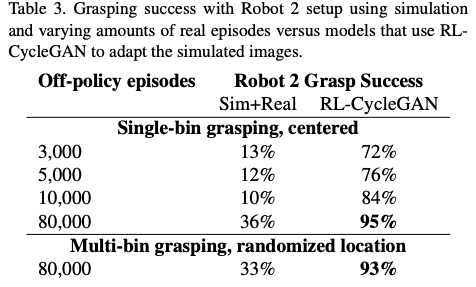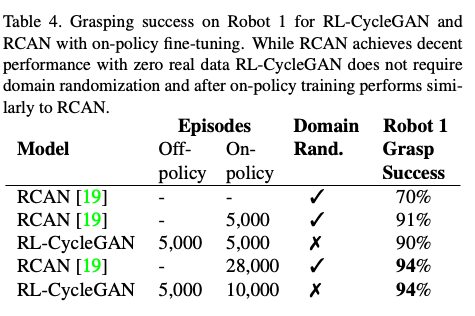# Catch up on the latest AI articles# Sim2real Transfer With CycleGan Integrated Into RL

3 main points
✔️ Proposal of RL-CycleGAN, a new sim2real method
✔️ RL-scene consistency loss allows the generation of images while retaining task information.
✔️ Achieved a high success rate in object-grabbing tasks

RL-CycleGAN: Reinforcement Learning Aware Simulation-To-Real
written by Kanishka RaoChris HarrisAlex IrpanSergey LevineJulian IbarzMohi Khansari
(Submitted on 16 June 2020)

Comments: Proceedings of the IEEE Conference on Computer Vision and Pattern Recognition (CVPR 2020)
Subjects: Robotics (cs.RO); Computer Vision and Pattern Recognition (cs.CV); Machine Learning (cs.LG)
Paper
Official Code COMM Code

## Introduction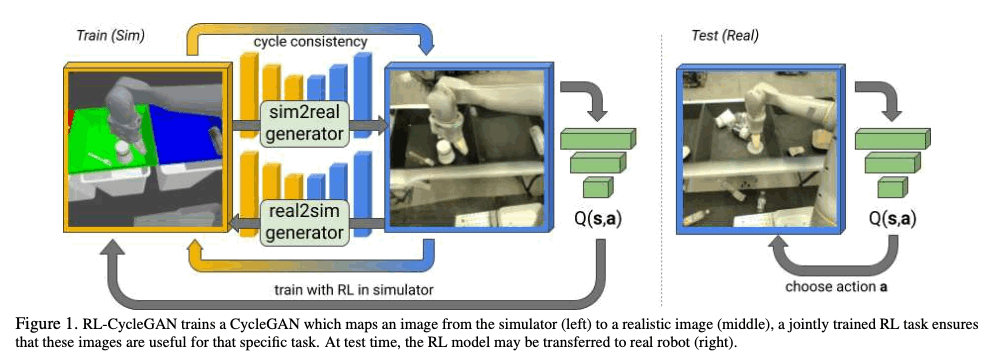## Technique

### CycleGAN

We begin by introducing CycleGAN, an important base model in the proposed method. CycleGAN is a method for learning from unpaired data $\left\{x_{i}\right\}_{i=1}^{N} \in X$ and $\left\{y_{i}\right\}_{i=1}^{M} \in Y$ with respect to the mapping of two different domains $X$ and $Y$. CycleGAN in Sim2Real can be represented by the domains $X$ and $Y$ as simulation and reality, respectively. CycleGAN consists of two generators, Sim2Real $G: X \rightarrow Y$ and Real2Sim $F: Y\rightarrow X$. Then, out of the two discriminators, $D_{X}$ identifies the simulation image $\{x\}$ and the image $\{G(y)\}$ generated based on the real image and $D_{Y}$ identifies the real image $\{y\}$ and the image $\{F(x)\}$ generated based on the simulation image. An adversarial loss is then applied to these two mappings, and the loss function of the GAN can be expressed as follows. \begin{aligned} \mathcal{L}_{G A N}\left(G, D_{Y}, X, Y\right)=& \mathbb{E}_{y \sim Y}\left[\log D_{Y}(y)\right] \\ &+\mathbb{E}_{x \sim X}\left[\log \left(1-D_{Y}(G(x))\right)\right] \end{aligned}

And then, CycleGAN adds the following loss function so that the relationship between $x \rightarrow G(x) \rightarrow F(G(x)) \approx x$ and $y \rightarrow F(y) \rightarrow G(F(y)) \approx y$ holds.
\begin{aligned} \mathcal{L}_{c y c}(G, F)=& \mathbb{E}_{x \sim \mathcal{D}_{s i m}} d(F(G(x)), x) \\ &+\mathbb{E}_{y \sim \mathcal{D}_{r e a l}} d(G(F(y)), y) \end{aligned}
Here, d represents the distance function, and in this paper, the mean square error was used.

### RL-CycleGAN

The important point in this paper is to retain information about the RL task when transforming images from simulation to real. For example, it is possible to generate realistic images by using conventional CycleGAN, but if it is difficult to transform an object, there is a possibility that the details of the object will be lost. Since the output of the RL model should depend on the semantics of the task, the GAN can be constrained by using the RL model to generate The goal is for the GAN to retain task-specific information by applying

For RL tasks the deep Q-learning network $Q(s, a)$ is used, where $s$ is the input image and $a$ is the action. In RL-CycleGAN, Q-functions are trained for both simulation and real, represented as $Q_{sim}(s, a)$ and $Q_{real}(s, a)$, respectively. In RL-CycleGAN, the RL model and CycleGAN are trained simultaneously, and the six input images $\{x, G(x), F(G(x))\}$ and $\{y, F(y), G(F(y))\}$ are passed to $Q_{sim}$ and $Q_{real}$, and the following six Q-values are calculated.

$$\begin{equation*}\begin{split}{c}(x, a) \sim \mathcal{D}_{\operatorname{sim}},(y, a) \sim \mathcal{D}_{\text {real}}q_{x}&=Q_{\operatorname{sim}}(x, a)q_{x}^{\prime}\\&=Q_{\text {real}}(G(x), a)q_{x}^{\prime \prime}\\&=Q_{\operatorname{sim}}(F(G(x)), a)q_{y}\\&=Q_{\text {real}}(y, a)q_{y}^{\prime}\\&=Q_{\operatorname{sim}}(F(y), a)q_{y}^{\prime \prime}\\&=Q_{\text {real}}(G(F(y)), a)\end{split}\end{equation*}$$

Here, $\{x, G(x), F(G(x))\}$, $\{y, F(y), G(F(y))\}$, $Q_{sim}$, and $Q_{real}$ each represent the same image, so the Q-values that take them as input must be similar. To facilitate this, the following loss function, called RL-scene consistency loss, is used.

\begin{aligned} \mathcal{L}_{R L-\text {scene}}(G, F)=& d\left(q_{x}, q_{x}^{\prime}\right)+d\left(q_{x}, q_{x}^{\prime \prime}\right)+d\left(q_{x}^{\prime}, q_{x}^{\prime \prime}\right)+d\left(q_{y}, q_{y}^{\prime}\right)+d\left(q_{y}, q_{y}^{\prime \prime}\right)+d\left(q_{y}^{\prime}, q_{y}^{\prime \prime}\right) \end{aligned}

Because the simulation and real images can be very different, instead of using one Q-network, we train a separate Q-network for simulation and real, where d is the distance function and the mean square error. This Q-network is trained using the following loss function, which is the usual TD-loss function.

$$\mathcal{L}_{R L}(Q)=\mathbb{E}_{\left(x, a, r, x^{\prime}\right)} d\left(Q(x, a), r+\gamma V\left(x^{\prime}\right)\right)$$

By combining all these loss functions together, we can finally represent the loss function of RL-CycleGAN with the following function.

$$\begin{array}{l} \mathcal{L}_{R L}-C y c l e G A N\left(G, F, D_{X}, D_{Y}, Q\right)&=\lambda_{G A N} \mathcal{L}_{G A N}\left(G, D_{Y}\right)\\&+\lambda_{G A N} \mathcal{L}_{G A N}\left(F, D_{X}\right)+\lambda_{c y c l e} \mathcal{L}_{c y c}(G, F)\quad\\&+\lambda_{R L-s c e n c e} \mathcal{L}_{R L-s c e n e}(G, F)+\lambda_{R L} \mathcal{L}_{R L}(Q) \end{array}$$

The image below shows the RL-CycleGAN models. All models are trained using the distributed Q-learning QT-Opt algorithm. Then, after RL-CycleGAN is trained, $Q_{real}$ is used for the policy to run the real-world robot.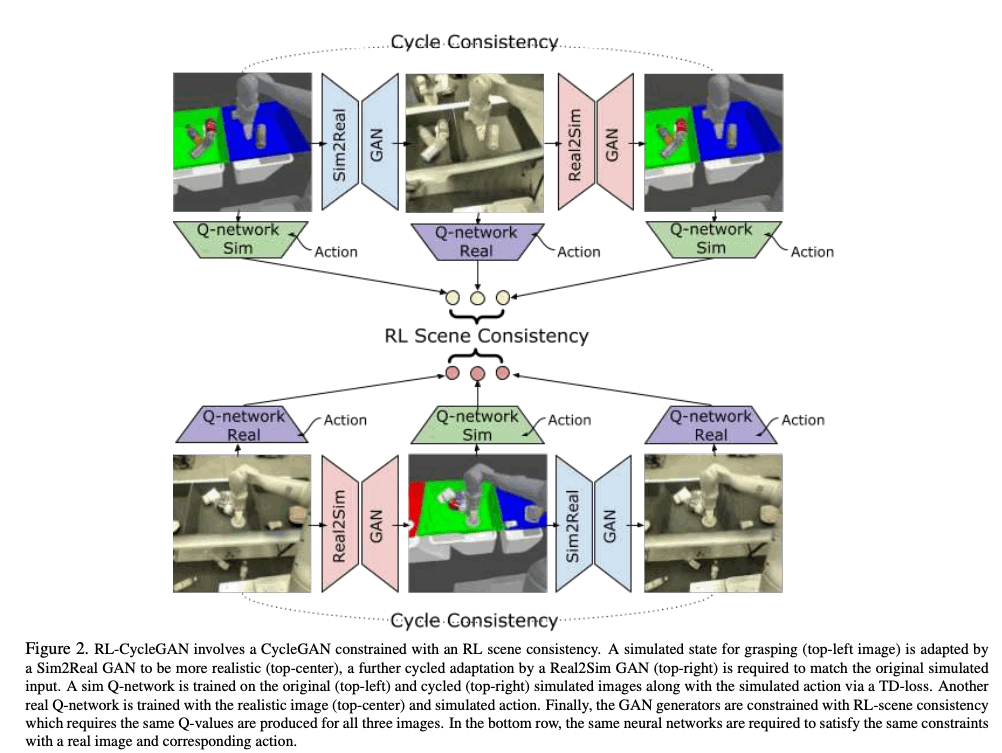## Experiment

Two tasks are considered in the experiments in this paper. In this experiment, we use a robot called Kuka IIWA to challenge ourselves with respect to the task of grasping various objects. In this section, we will introduce the two tasks performed in this paper.

Robot 1 Setup: This task evaluates the ability of the robot to grab six unknown objects in a bin, after training the model to grab various objects in the bin. The data of the real-world robot to train the model is collected by using the already trained model or by writing a script. As shown in the image below, simulations perform poorly when using simulated policies on real-world robots because the environment is not very realistic.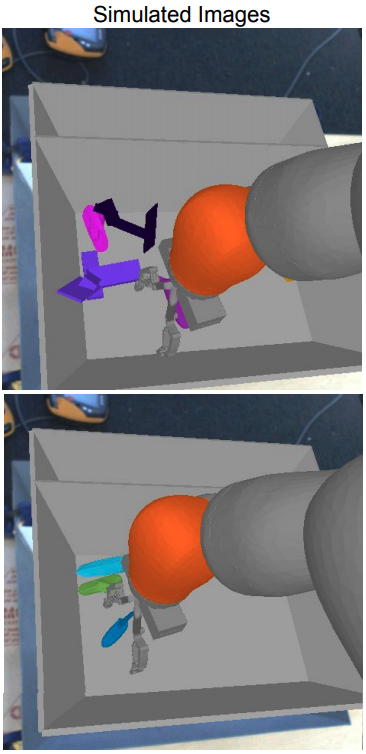Robot 2 Setup: In the second task, we experiment with three adjacent bins, as shown in the following image. In this experiment, the robot is randomly placed in front of a bin, and we investigate the generalization performance with respect to position and camera angle by checking whether the robot can grab an object from any bin. In this task, single-bin grasping and multi-bin grasping are evaluated in two ways, as shown in the following figure, where single-bin grasping evaluates the success rate of a robot fixed in the central bin, whereas multi-bin The grasping evaluates the success rate of a robot randomly placed before bin.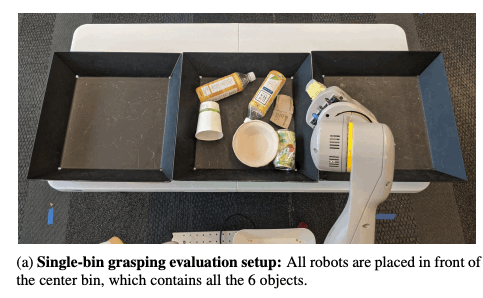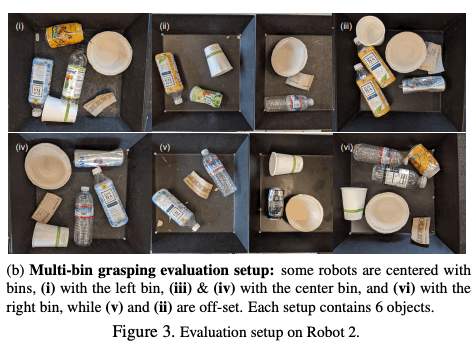### Results for Robot 1

The table below shows an evaluation of the task for Robot 1, where each method is compared in terms of its success in grabbing an unknown object. First, we present a few baselines to evaluate the proposed method in this paper: the first is the use of a policy learned only in simulation without adaptation to a real-world robot. As the table below shows, this has a very low success rate of 21%, indicating a large gap between simulation and real. The next one, called Randomized Sim, increases the generalization performance for unknown image inputs by randomly changing the images of the robot's arm, object, bin, and background. This method has a higher success rate than Sim-Only, but it remains low. Another baseline is to learn the mapping from simulation to real using several GANs, and to learn the policy using the GAN transformations of the images from simulation. The results show that RL-CycleGAN has a higher success rate than the other methods. As you can see from the transformed images below, RL-CycleGAN is able to transform the important parts of the task, while the other methods generate images with the important objects disappearing. This shows that RL-CycleGAN is able to generate images while retaining the information that is important to the task.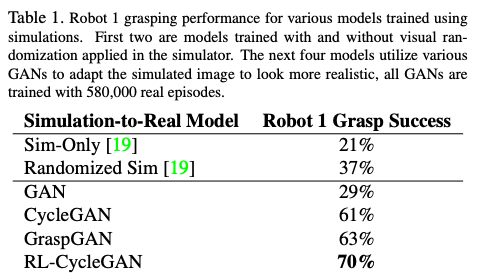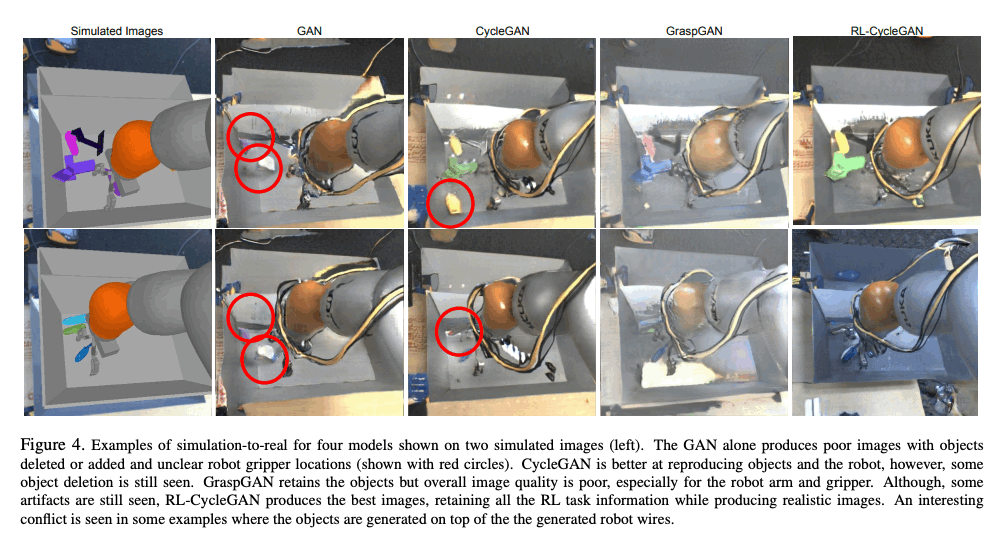### The relationship between the amount of real data and performance

In the experiments in the previous section, we used real data only for GAN training, and in RL training, we used simulation data converted through GAN. In this experiment, we investigated how the success rate of the object-grabbing task changes as the amount of real training data increases when real data is also used for RL training, i.e., both real off-policy data and on-policy data transformed through the GAN are used for training. We compared the tasks for Robot 1 and Robot 2. The table below shows the results for the Robot 1 task, and we can see that as we have more real data, our success rate has improved significantly.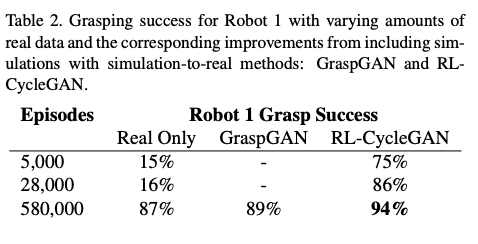The table below shows the results of the experiments on the Robot 2 task and shows that using RL-CycleGAN, even with a small number of data (3000 episodes of data), the success rate ranged from 17% to 72%, with no adaptation to simulate and real data. We can see that the success rate has increased significantly compared to the case of just using it. And similar to the results for the Robot 1 task, we can see that increasing the number of data increases the success rate significantly when using RL-CycleGAN. Also, when comparing single-bin and multi-bin grasping results, the success rate per the same number of real data is almost the same, indicating that there is good generalization performance for different locations and camera angles.